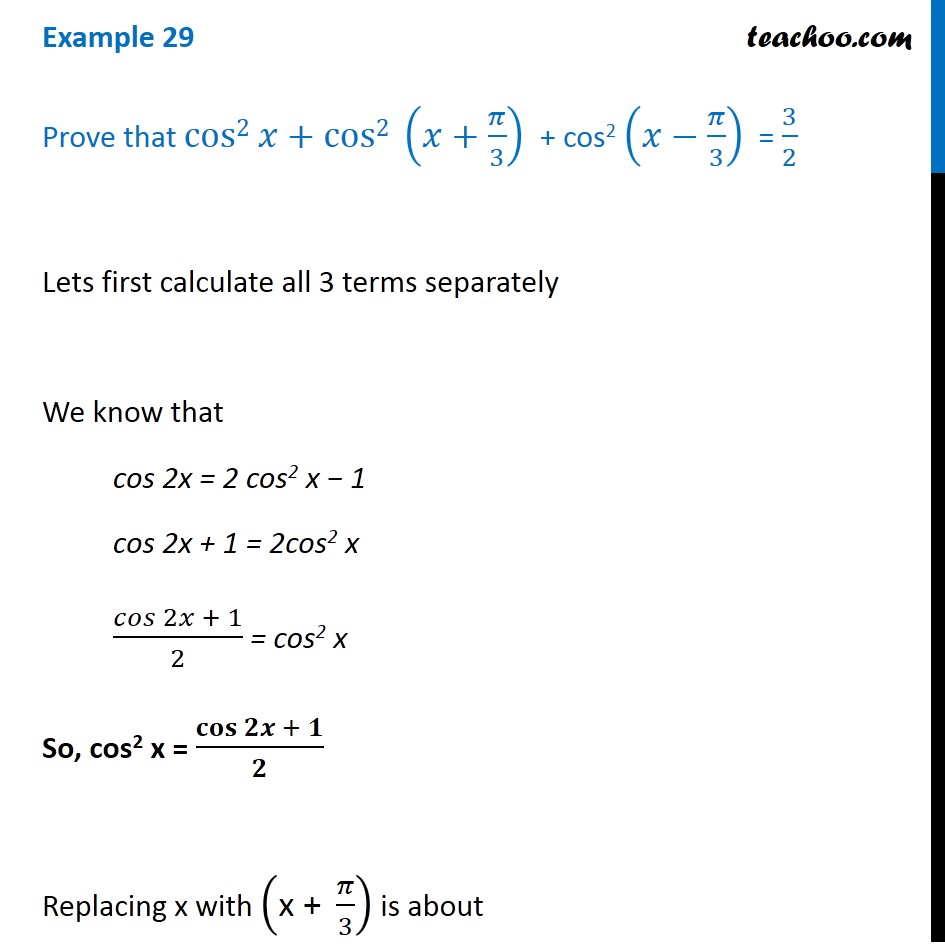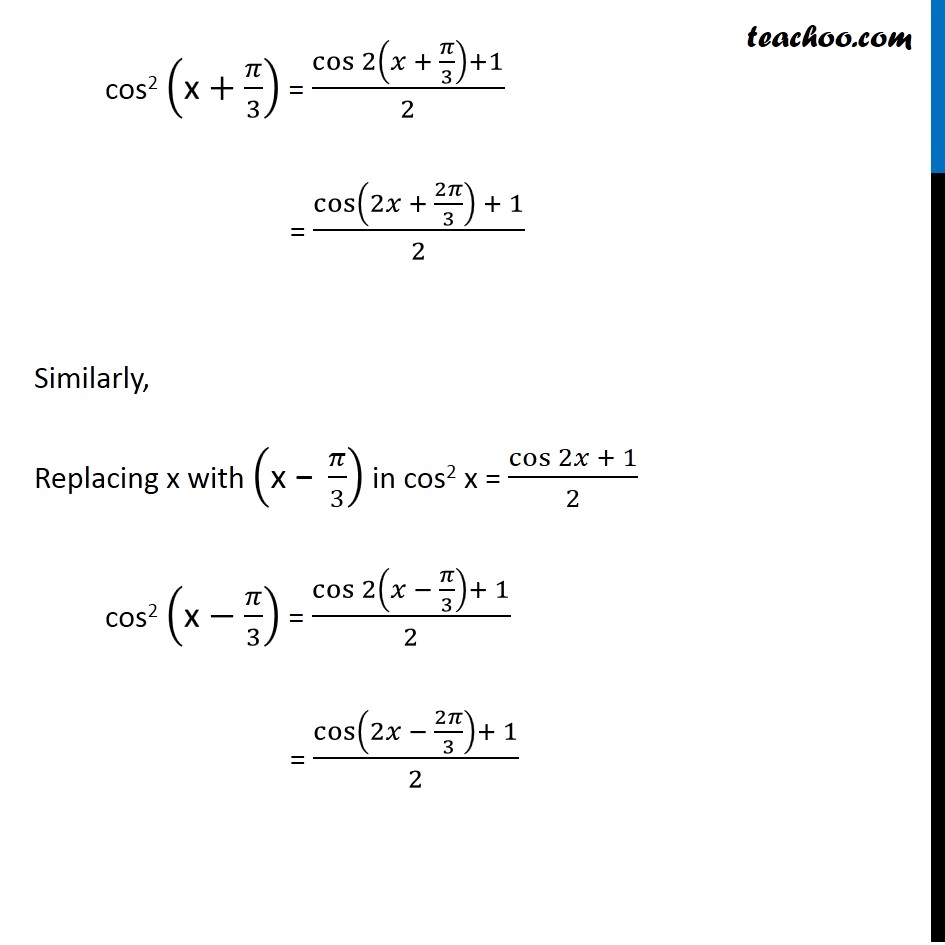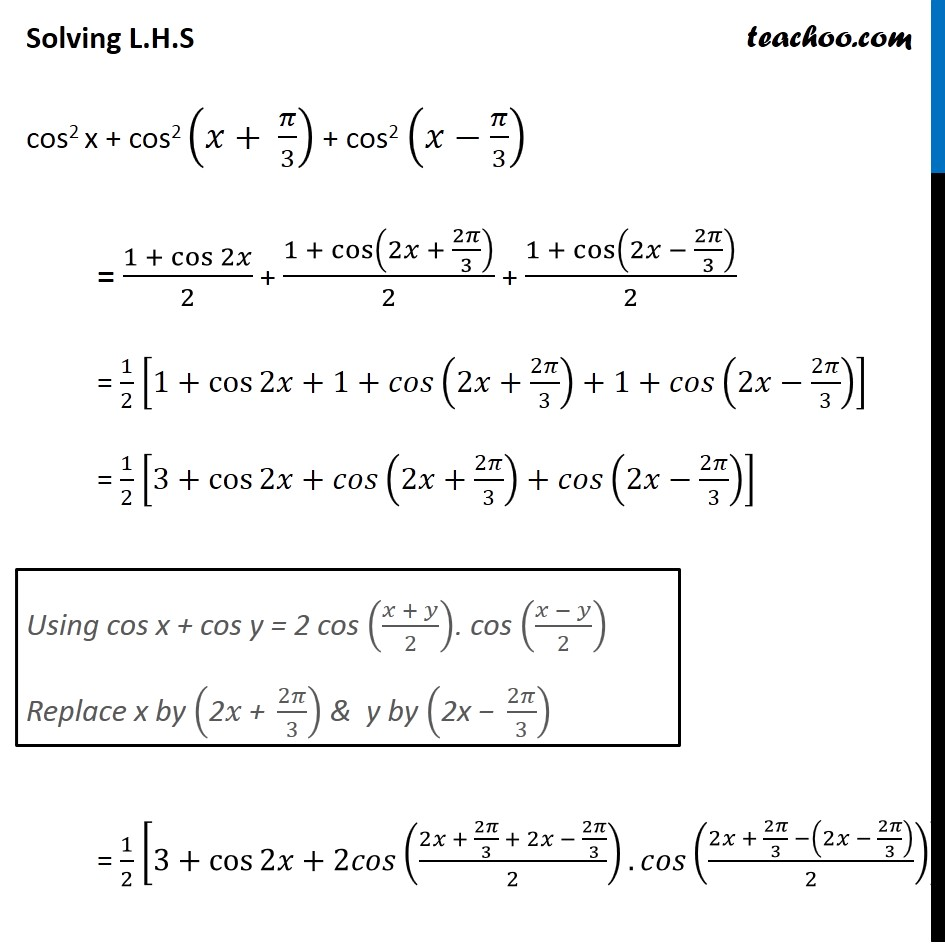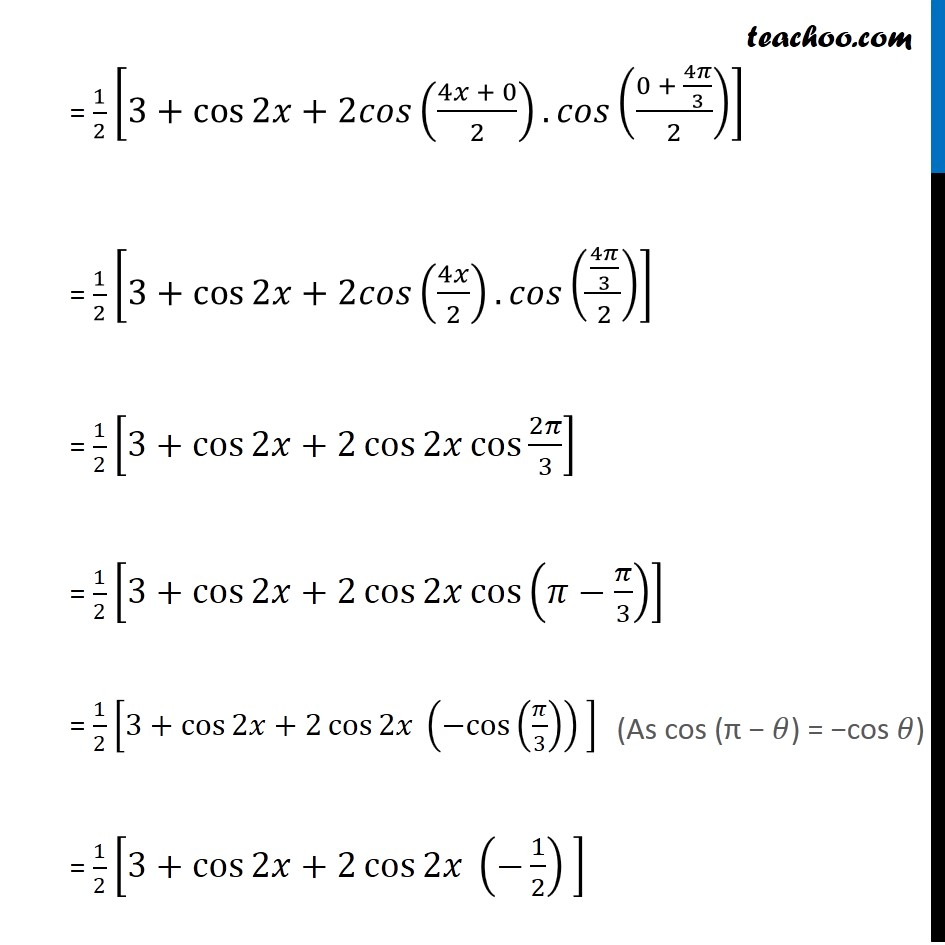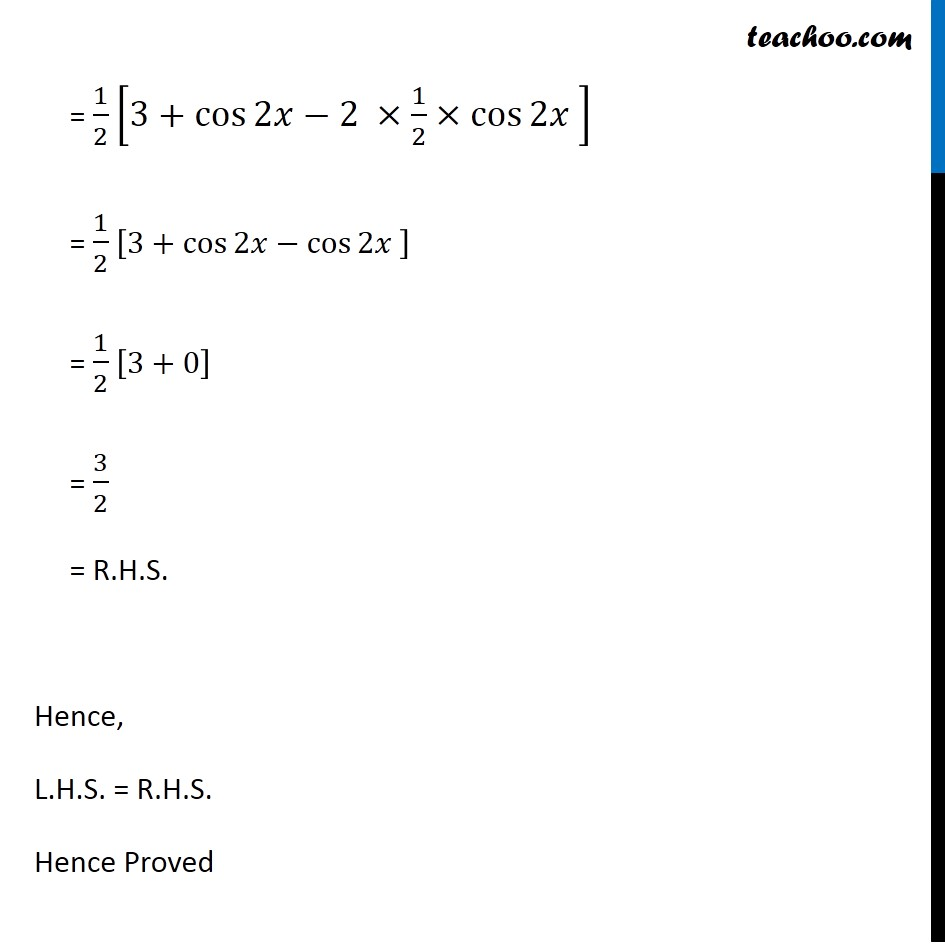1. Chapter 3 Class 11 Trigonometric Functions (Term 2)
2. Concept wise
3. 2x 3x formula - Proving

Transcript

Example 29 Prove that cos2 𝑥+cos2 (𝑥+𝜋/3) + cos2 (𝑥−𝜋/3) = 3/2 Lets first calculate all 3 terms separately We know that cos 2x = 2 cos2 x − 1 cos 2x + 1 = 2cos2 x 𝑐𝑜𝑠⁡〖2𝑥 + 1〗/2 = cos2 x So, cos2 x = 𝐜𝐨𝐬⁡〖𝟐𝒙 + 𝟏〗/𝟐 Replacing x with ("x + " 𝜋/3) is about cos2 ("x" +𝜋/3) = cos⁡〖2(𝑥 + 𝜋/3)+1〗/2 = cos⁡〖(2𝑥 + 2𝜋/3) + 1〗/2 Similarly, Replacing x with ("x −" 𝜋/3) in cos2 x = cos⁡〖2𝑥 + 1〗/2 cos2 ("x" −𝜋/3) = cos⁡〖2(𝑥 − 𝜋/3)+ 1〗/2 = cos⁡〖(2𝑥 − 2𝜋/3)+ 1〗/2 Solving L.H.S cos2 x + cos2 (𝑥+ 𝜋/3) + cos2 (𝑥−𝜋/3) = (1 + cos⁡2𝑥)/2 + (1 + cos⁡(2𝑥 + 2𝜋/3))/2 + (1 + cos⁡(2𝑥 − 2𝜋/3))/2 = 1/2 [1+cos⁡〖2𝑥+1+𝑐𝑜𝑠(2𝑥+2𝜋/3)+1+𝑐𝑜𝑠(2𝑥−2𝜋/3)〗 ] = 1/2 [3+cos⁡〖2𝑥+𝑐𝑜𝑠(2𝑥+2𝜋/3)+𝑐𝑜𝑠(2𝑥−2𝜋/3)〗 ] = 1/2 [3+cos⁡〖2𝑥+2𝑐𝑜𝑠((2𝑥 + 2𝜋/3 + 2𝑥 − 2𝜋/3)/2).𝑐𝑜𝑠((2𝑥 + 2𝜋/3 −(2𝑥 − 2𝜋/3))/2)〗 ] Using cos x + cos y = 2 cos ((𝑥 + 𝑦)/2). cos ((𝑥 − 𝑦)/2) Replace x by ("2" 𝑥" + " 2𝜋/3) & y by ("2x − " 2𝜋/3) = 1/2 [3+cos⁡〖2𝑥+2𝑐𝑜𝑠((4𝑥 + 0)/2).𝑐𝑜𝑠((0 + 4𝜋/3)/2)〗 ] = 1/2 [3+cos⁡〖2𝑥+2𝑐𝑜𝑠(4𝑥/2).𝑐𝑜𝑠((4𝜋/3)/2)〗 ] = 1/2 [3+cos⁡〖2𝑥+2 cos⁡2𝑥 cos⁡〖2𝜋/3〗 〗 ] = 1/2 [3+cos⁡〖2𝑥+2 cos⁡2𝑥 cos⁡(𝜋−𝜋/3) 〗 ] = 1/2 [3+cos⁡〖2𝑥+2 cos⁡2𝑥 〗 (〖−cos〗⁡(𝜋/3) ) ] = 1/2 [3+cos⁡〖2𝑥+2 cos⁡2𝑥 〗 (−1/2) ] (As cos (π − 𝜃) = −cos 𝜃) = 1/2 [3+cos⁡〖2𝑥−2 ×1/2×cos⁡2𝑥 〗 ] = 1/2 [3+cos⁡〖2𝑥−cos⁡2𝑥 〗 ] = 1/2 [3+0] = 3/2 = R.H.S. Hence, L.H.S. = R.H.S. Hence Proved